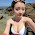Sunday, September 20, 2015

NJournal Notes 2.0

https://en.wikipedia.org/wiki/Multifractal_system

multifractal system is a generalization of a fractal system in which a single exponent (thefractal dimension) is not enough to describe its dynamics; instead, a continuous spectrum of exponents (the so-called singularity spectrum) is needed.
Multifractal systems are common in nature, especially geophysics. They include fully developed turbulencestock market time series, real world scenes, the Sun’s magnetic field time series, heartbeat dynamics, human gait, and natural luminosity time series. Models have been proposed in various contexts ranging from turbulence in fluid dynamics to internet traffic, finance, image modeling, texture synthesis, meteorology, geophysics and more. The origin of multifractality in sequential (time series) data has been attributed to mathematical convergence effects related to the central limit theorem that have as foci of convergence the family of statistical distributions known as the Tweedie exponential dispersion modelsas well as the geometric Tweedie models. The first convergence effect yields monofractal sequences and the second convergence effect is responsible for variation in the fractal dimension of the monofractal sequences.

From a practical perspective, multifractal analysis uses the mathematical basis of multifractal theory to investigate datasets, often in conjunction with other methods offractal analysis and lacunarity analysis. The technique entails distorting datasets extracted from patterns to generate multifractal spectra that illustrate how scaling varies over the dataset. The techniques of multifractal analysis have been applied in a variety of practical situations such as predicting earthquakes and interpreting medical images.

1.2.3.